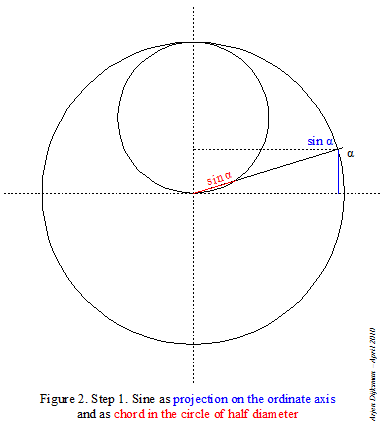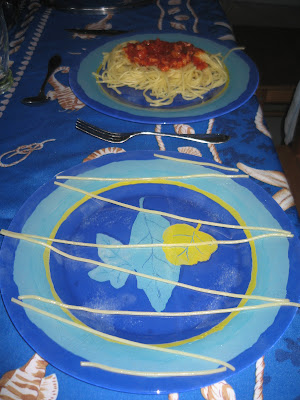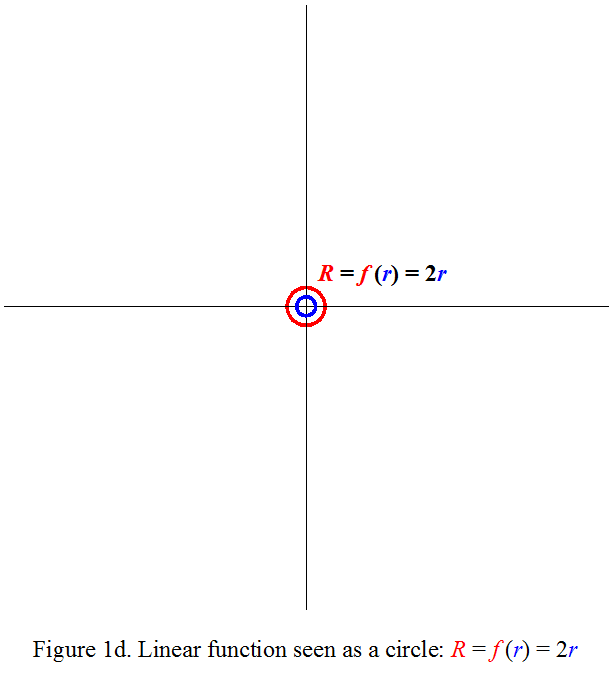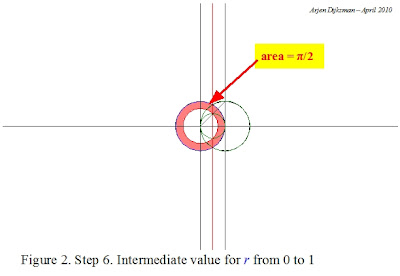## Sunday, April 11, 2010

### Teaching sine function with spaghetti

Sine functions appear everywhere in physics and mathematics. This seems to be related to the circular symmetry of space and to the periodic behavior of dynamical processes. The usual definition is that the sine of an angle is the ratio of the length of the opposite side to the length of the hypotenuse in a right triangle (definition at wikipedia). The sine is also often represented as the ordinate of a point running on a unit circle centered at the origin. Any right triangle can be put in that setting. The length of the blue segment on Figure 1 illustrates this definition for an arbitrary angle α, in a circle of unit radius.

It is of interest to have in mind other geometric representations of the sine function. This helps to associate figures with sine and cosine operations or identities. The red segment in Figure 1 shows such an alternative representation, because the sine is also a chord of a circle of unit diameter. When we increment the angle by steps α, one endpoint of the chord advances on the little circle, while the other endpoint remains fixed at the origin, as illustrated by the animation in Figure 2.When it represents the sine of angle α, the chord in the little circle spans an angle 2α. It is therefore a direct way to the angle multiplication and division by 2. Moreover, the endpoints of this chord can be placed two by two in any other direction on this circle. This enables one to find other variations on the recurrence pattern of the angle in the circle. During one of my circle drawing sessions, I was surprised by the stepwise zigzagging pattern of Figure 3.

Teaching the sine function with this zigzag pattern is particularly suited for daily life situations. Children like it, especially when you explain it at the table.## Monday, April 5, 2010

### Keeping track of the circle for integral representations of π

The fundamental constant π is characterized in many ways. Historically, it all began from tentative measurements of the circle's perimeter or area and gradually shifted into more advanced mathematics, in such a way that the link between the circle and modern characterizations of π faded away, see for example the formulas presented at Wolfram MathWorld or Wikipedia.

In the preceding posts, I mentioned infinite products as approximations for π. These may be seen geometrically as exhaustion methods, where the area of a polygon approaches the circular area alternately from above, from below, from above, from below, etc.
There are also integral representations of pi. In such integral representations, π appears in the quantitative value of the integral of a mathematical function. Visually, this is often represented as the area delimited by the bounds of the function. However, the relation with the circle is lost, when viewed under Cartesian coordinates. For example, the graph of the simplest instance of the Cauchy-Lorentz distribution, f(x)=1/(1+x²), "has nothing at all to do with circles or geometry in any obvious way" as quoted from last Pi-day Sunday function from Matt Springer's Built on Facts blog.In order to view the role of the circle in integral representations of π, we need to switch to alternative ways to visualize math functions. As an example, let's take the constant function y=f(x)=2. The function f maps an element x from a domain to the element y of the target. In this case, for every x, the target y has the constant value 2. With Cartesian coordinates, we are used to represent this function as a horizontal straight line, like in Figure 1a (click on the figure to view it enlarged). If however we write it as R=f(r)=2, where the function f maps any circle of radius r of the domain to a target circle of radius R=2, the same function can be viewed as a circle of constant radius, like in Figure 1b. So the same function f can be equally well viewed as a straight line or as a circle (x, y, r or R are only dummy variables).Now if we take another example, the linear function, y=f(x)=2x, we are often used to view it in Cartesian coordinates as a straight line with slope 2, like in Figure 1c. In the circular representation R=f(r)=2r, this works however differently. Because we are relating circles of the input domain to other circles of the target, for each circle of radius r, we need to draw the target circle of radius 2r. A single line won't do. For one value of r, we need to draw two circles. If we use blue circles for elements of the input domain and red circles for elements of the target, we could visualize it for successive values of r as an animation like in Figure 1d. In that way, we view the progression of the target circle as the input circle becomes larger.Unlike the Cartesian representation which shows the progression of a function in a static graph, this circular representation needs a dynamic or recurrent process to get grip of the progression of the function. Therefore it isn't very adapted for illustrations in print media. On the other hand, it has the advantage of keeping track of the geometrical form of the circle. And that's exactly what we need in order to perceive the circular nature when π shows up in mathematical functions. The relation of the integral of the Cauchy-Lorentz distribution f(r)=1/(1+r²) with the circle can then be seen with the help of the geometric counterparts of arithmetic operations like addition, squaring and dividing. A convenient procedure is illustrated in the successive steps of Figure 2.

Step 1. Draw the input circle of radius r and the reference circle of radius unity.

Step 2. Determine r².Step 5. Find the target ring related to the input ring ranging over [r, r + dr]. This yields a ring of width dr/(1+r²). The location of this ring depends on the relative progression rates of r and r² (I've not yet found a straightforward explanation for this determination).Step 6. Integrate dr/(1+r²) for r running over all space. For r becoming larger and larger, the summed area tends towards the area of a circle of radius 1. For the positive half plane, this corresponds to the π/2 value found analytically.

The tricky step seems to be the way how to relate the progression between r and 1/(1+r²) in steps 5 and 6. One can verify for example the value of the integral at intermediate steps. For the integral from r=0 to 1, the value in the positive half plane must be π/4, which can be verified on the figure below.In order to gain more insight on π, it could be of interest to develop skills for this circular representation.